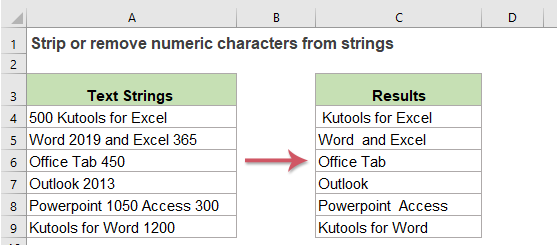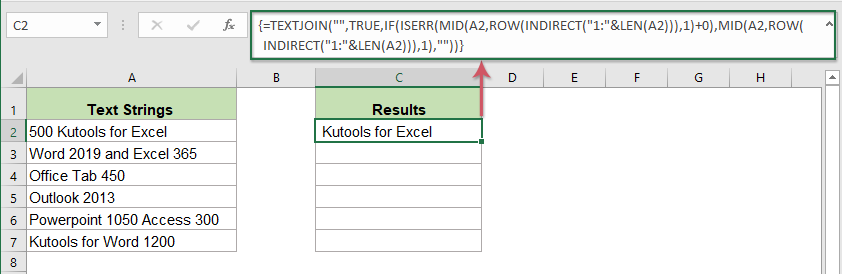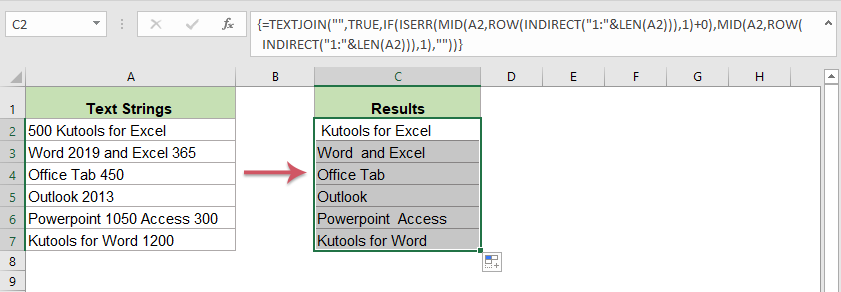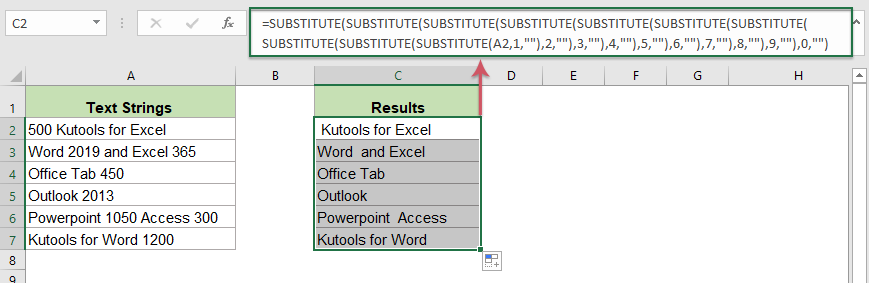## Strip or remove numeric characters from text strings

If you want to remove all numbers only from a list of text strings but keep other non-numeric characters, maybe there are some formulas in Excel can do you a favor.#### Strip or remove all numbers from text strings in Excel

To remove only numbers from alphanumeric strings, a complex array formula which combines the TEXTJOIN, MID, ROW, IF, ISERR, LEN and the INDIRECT functions can help you. (Note: This formula only works well in Excel 2019 and Office 365.) The generic syntax is:

=TEXTJOIN("",TRUE,IF(ISERR(MID(text,ROW(INDIRECT("1:"&LEN(text))),1)+0),MID(text,ROW(INDIRECT("1:"&LEN(text))),1),""))
• text: the text string or cell value that you want to remove all numbers from.

1. Please copy or enter the below formula into a blank cell where you want to output the result:

=TEXTJOIN("",TRUE,IF(ISERR(MID(A2,ROW(INDIRECT("1:"&LEN(A2))),1)+0),MID(A2,ROW(INDIRECT("1:"&LEN(A2))),1),""))

2. Then, press Ctrl + Shift + Enter keys simultaneously to get the first result, see screenshot:3. And then, select the formula cell, drag the fill handle down to the cells that you want to use, all the numbers have been stripped from the text strings, see screenshot:##### Explanation of the formula:

ROW(INDIRECT("1:"&LEN(A2))): The INDIRECT function within the ROW function will return an array list which length is the length of cell A2 like the below, and this part is recognized as the start_num in the MID function.
{1;2;3;4;5;6;7;8;9;10;11;12;13;14;15;16;17;18;19;20;21}

MID(A2,ROW(INDIRECT("1:"&LEN(A2))),1): This MID function is used to extract text from cell A2 to get one character, and it will return array like this:
{ "5";"0";"0";" ";"K";"u";"t";"o";"o";"l";"s";" ";"f";"o";"r";" ";"E";"x";"c";"e";"l"}

MID(A2,ROW(INDIRECT("1:"&LEN(A2))),1)+0: Adding 0 value after this array is used to force the text to a number, the numeric text value will be converted to number, and non-numeric values will be displayed as #VALUE error value like this:
{"5";"0";"0";#VALUE!;#VALUE!;#VALUE!;#VALUE!;#VALUE!;#VALUE! !;#VALUE! !;#VALUE!...}

IF(ISERR(MID(A2,ROW(INDIRECT("1:"&LEN(A2))),1)+0),MID(A2,ROW(INDIRECT("1:"&LEN(A2))),1),""): The IF function is used to check if the array returned by MID function are error values or not. If it is an error value, then get the non-numeric character using the second MID function, otherwise, empty strings are displayed like this:
{ "";"";"";" ";"K";"u";"t";"o";"o";"l";"s";" ";"f";"o";"r";" ";"E";"x";"c";"e";"l"}

TEXTJOIN("",TRUE,IF(ISERR(MID(A2,ROW(INDIRECT("1:"&LEN(A2))),1)+0),MID(A2,ROW(INDIRECT("1:"&LEN(A2))),1),"")): Finally, the TEXTJOIN function will join all values and ignore the empty string to get the result.

##### Notes:

1. When removing numeric characters, maybe there are some extra space characters left, to remove all extra spaces, you should wrap the formula into the TRIM function as below:

=TRIM(TEXTJOIN("",TRUE,IF(ISERR(MID(A2,ROW(INDIRECT("1:"&LEN(A2))),1)+0),MID(A2,ROW(INDIRECT("1:"&LEN(A2))),1),"")))

2. To remove numbers from text strings in Excel early versions, please apply the following formula:

=SUBSTITUTE(SUBSTITUTE(SUBSTITUTE(SUBSTITUTE(SUBSTITUTE(SUBSTITUTE(SUBSTITUTE(SUBSTITUTE(SUBSTITUTE(SUBSTITUTE(A2,1,""),2,""),3,""),4,""),5,""),6,""),7,""),8,""),9,""),0,"")#### Relative functions used:

• TEXTJOIN:
• The TEXTJOIN function joins multiple values from a row, column or a range of cells with specific delimiter.
• MID:
• The MID function is used to find and return a specific number of characters from the middle of given text string.
• ROW:
• The Excel ROW function returns the row number of a reference.
• INDIRECT:
• The Excel INDIRECT function converts a text string to a valid reference.
• LEN:
• The LEN function returns the number of characters in a text string.
• IF:
• IF function performs a simple logical test which depending on the comparison result, and it returns one value if a result is TRUE, or another value if result is FALSE.
• ISERR:
• The ISERR function is used to return TRUE for any error type except the #N/A error.

#### More articles:

• Remove Line Breaks From Cells In Excel
• This tutorial provides three formulas to help you removing line breaks (which are occurred by pressing Alt + Enter keys in a cell) from specific cells in Excel.

### The Best Office Productivity Tools

#### Kutools for Excel - Helps You To Stand Out From Crowd

 Popular Features: Find, Highlight or Identify Duplicates  |  Delete Blank Rows  |  Combine Columns or Cells without Losing Data  |  Round without Formula ... Super VLookup: Multiple Criteria  |  Multiple Value  |  Across Multi-Sheets  |  Fuzzy Lookup... Adv. Drop-down List: Easy Drop Down List  |  Dependent Drop Down List  |  Multi-select Drop Down List... Column Manager: Add a Specific Number of Columns  |  Move Columns  |  Toggle Visibility Status of Hidden Columns  |  Compare Columns to Select Same & Different Cells ... Featured Features: Grid Focus  |  Design View  |  Big Formula Bar  |  Workbook & Sheet Manager | Resource Library (Auto Text)  |  Date Picker  |  Combine Worksheets  |  Encrypt/Decrypt Cells  |  Send Emails by List  |  Super Filter  |  Special Filter (filter bold/italic/strikethrough...) ... Top 15 Toolsets:  12 Text Tools (Add Text, Remove Characters ...)  |  50+ Chart Types (Gantt Chart ...)  |  40+ Practical Formulas (Calculate age based on birthday ...)  |  19 Insertion Tools (Insert QR Code, Insert Picture from Path ...)  |  12 Conversion Tools (Numbers to Words, Currency Conversion ...)  |  7 Merge & Split Tools (Advanced Combine Rows, Split Excel Cells ...)  |  ... and more

Kutools for Excel Boasts Over 300 Features, Ensuring That What You Need is Just A Click Away...#### Office Tab - Enable Tabbed Reading and Editing in Microsoft Office (include Excel)

• One second to switch between dozens of open documents!
• Reduce hundreds of mouse clicks for you every day, say goodbye to mouse hand.
• Increases your productivity by 50% when viewing and editing multiple documents.
• Brings Efficient Tabs to Office (include Excel), Just Like Chrome, Edge and Firefox.